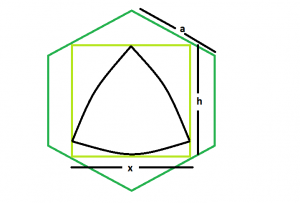# Biggest Reuleaux Triangle inscribed within a square which is inscribed within a hexagon

Given a regular hexagon of side length a which inscribes a square which in turn inscribes a reuleaux triangle. The task is to find the maximum possible area of this reuleaux triangle.

Examples:

```Input: a = 5
Output: 28.3287

Input: a = 9
Output: 91.7848
```## Recommended: Please try your approach on {IDE} first, before moving on to the solution.

Approach: As the side of the square inscribed within a hexagon is x = 1.268a. Please refer Largest Square that can be inscribed within a hexagon.
Also, in the reuleaux triangle, h = x = 1.268a.
So, Area of the reuleaux triangle, A = 0.70477*h^2 = 0.70477*(1.268a)^2.

Below is the implementation of the above approach:

## C++

 `// C++ Program to find the biggest Reuleaux triangle ` `// inscribed within in a square which in turn ` `// is inscribed within a hexagon ` `#include ` `using` `namespace` `std; ` ` `  `// Function to find the biggest reuleaux triangle ` `float` `Area(``float` `a) ` `{ ` ` `  `    ``// side cannot be negative ` `    ``if` `(a < 0) ` `        ``return` `-1; ` ` `  `    ``// height of the reuleaux triangle ` `    ``float` `h = 1.268 * a; ` ` `  `    ``// area of the reuleaux triangle ` `    ``float` `A = 0.70477 * ``pow``(h, 2); ` ` `  `    ``return` `A; ` `} ` ` `  `// Driver code ` `int` `main() ` `{ ` `    ``float` `a = 5; ` `    ``cout << Area(a) << endl; ` ` `  `    ``return` `0; ` `} `

## Java

 `// Java Program to find the biggest Reuleaux triangle ` `// inscribed within in a square which in turn ` `// is inscribed within a hexagon ` ` `  `import` `java.io.*; ` ` `  `class` `GFG  ` `{ ` ` `  `// Function to find the biggest reuleaux triangle ` `static` `float` `Area(``float` `a) ` `{ ` ` `  `    ``// side cannot be negative ` `    ``if` `(a < ``0``) ` `        ``return` `-``1``; ` ` `  `    ``// height of the reuleaux triangle ` `    ``float` `h =(``float``) ``1.268` `* a; ` ` `  `    ``// area of the reuleaux triangle ` `    ``float` `A = (``float``)(``0.70477` `* Math.pow(h, ``2``)); ` ` `  `    ``return` `A; ` `} ` ` `  `    ``// Driver code ` `    ``public` `static` `void` `main (String[] args)  ` `    ``{ ` `        ``float` `a = ``5``; ` `    ``System.out.println( Area(a)); ` `    ``} ` `} ` ` `  `// This code is contributed by anuj_67 `

## Python3

 `# Python3 Program to find the biggest  ` `# Reuleaux triangle inscribed within ` `# in a square which in turn is ` `# inscribed within a hexagon ` `import` `math ` ` `  `# Function to find the biggest  ` `# reuleaux triangle ` `def` `Area(a): ` ` `  `    ``# side cannot be negative ` `    ``if` `(a < ``0``): ` `        ``return` `-``1` ` `  `    ``# height of the reuleaux triangle ` `    ``h ``=` `1.268` `*` `a ` ` `  `    ``# area of the reuleaux triangle ` `    ``A ``=` `0.70477` `*` `math.``pow``(h, ``2``) ` ` `  `    ``return` `A ` ` `  `# Driver code ` `a ``=` `5` `print``(Area(a),end ``=` `"\n"``) ` ` `  `# This code is contributed  ` `# by Akanksha Rai `

## C#

 `// C# Program to find the biggest Reuleaux ` `// triangle inscribed within in a square  ` `// which in turn is inscribed within a hexagon ` `using` `System; ` ` `  `class` `GFG  ` `{ ` ` `  `// Function to find the biggest reuleaux triangle ` `static` `float` `Area(``float` `a) ` `{ ` ` `  `    ``// side cannot be negative ` `    ``if` `(a < 0) ` `        ``return` `-1; ` ` `  `    ``// height of the reuleaux triangle ` `    ``float` `h =(``float``) 1.268 * a; ` ` `  `    ``// area of the reuleaux triangle ` `    ``float` `A = (``float``)(0.70477 * Math.Pow(h, 2)); ` ` `  `    ``return` `A; ` `} ` ` `  `// Driver code ` `public` `static` `void` `Main ()  ` `{ ` `    ``float` `a = 5; ` `    ``Console.WriteLine(Area(a)); ` `} ` `} ` ` `  `// This code is contributed  ` `// by Akanksha Rai `

## PHP

 ` `

Output:

```28.3287
```

Attention reader! Don’t stop learning now. Get hold of all the important DSA concepts with the DSA Self Paced Course at a student-friendly price and become industry ready.

My Personal Notes arrow_drop_upBudding Web DeveloperKeen learnerAverage CoderDancer&Social Activist

If you like GeeksforGeeks and would like to contribute, you can also write an article using contribute.geeksforgeeks.org or mail your article to contribute@geeksforgeeks.org. See your article appearing on the GeeksforGeeks main page and help other Geeks.

Please Improve this article if you find anything incorrect by clicking on the "Improve Article" button below.

Improved By : vt_m, AnkitRai01, Akanksha_Rai